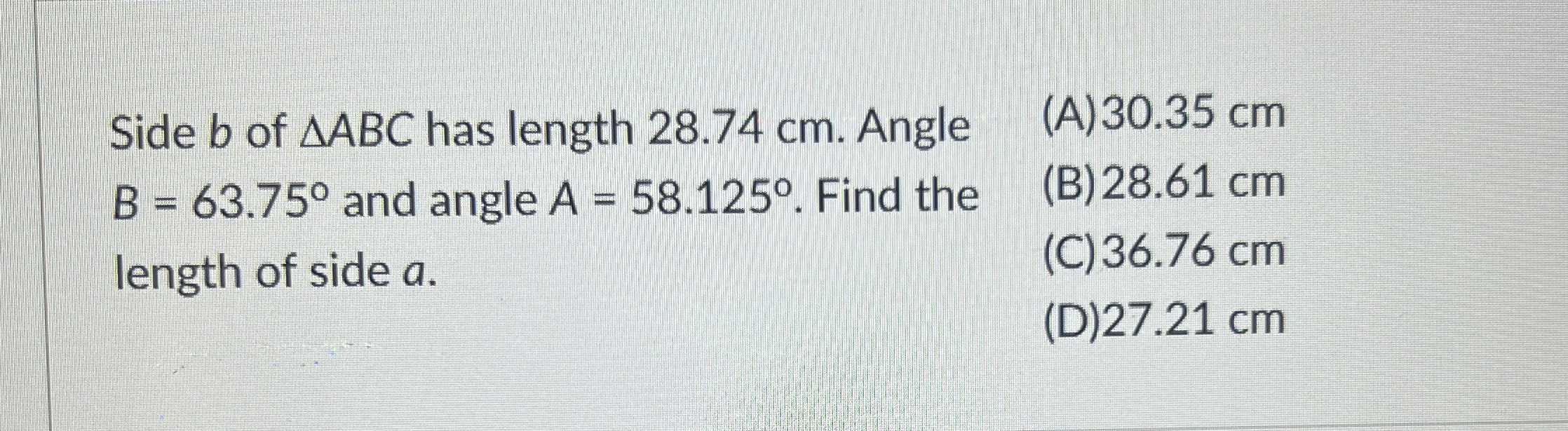### ¿Todavía tienes preguntas de matemáticas?

Pregunte a nuestros tutores expertos
Trigonometry
PreguntaSide $$b$$ of $$\triangle A B C$$ has length $$28.74 cm$$ . Angle

$$B = 63.75 ^ { \circ }$$ and angle $$A = 58.125 ^ { \circ } .$$ Find the length of side $$a .$$ (A) $$30.35 cm$$

(B) $$28.61 cm$$

(C) $$36.76 cm$$

(D) $$27.21 cm$$

(D) $$27.21 cm$$